## 1.2 词向量计算的工具——word2vec （有趣解释+代码）

2021/1/9 1:56:34 文章标签: word计算

https://github.com/graykode/nlp-tutorial

2013年，Google开源了一款用于词向量计算的工具——word2vec，引起了工业界和学术界的关注。首先，word2vec可以在百万数量级的词典和上亿的数据集上进行高效地训练；其次，该工具得到的训练结果——词向量（word embedding），可以很好地度量词与词之间的相似性。随着深度学习（Deep Learning）在自然语言处理中应用的普及，很多人误以为word2vec是一种深度学习算法。其实word2vec算法的背后是一个浅层神经网络。另外需要强调的一点是，word2vec是一个计算word vector的开源工具。当我们在说word2vec算法或模型的时候，其实指的是其背后用于计算word vector的CBoW模型和Skip-gram模型。

# 1.独热编码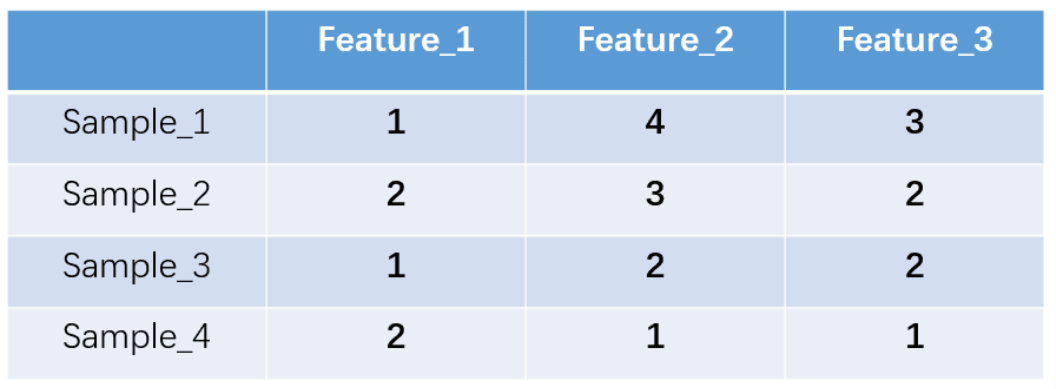我们的feature_1有两种可能的取值，比如是男/女，这里男用1表示，女用2表示。feature_2 和feature_3各有4种取值（状态）。one-hot编码就是保证每个样本中的单个特征只有1位处于状态1，其他的都是0。上述状态用one-hot编码如下图所示：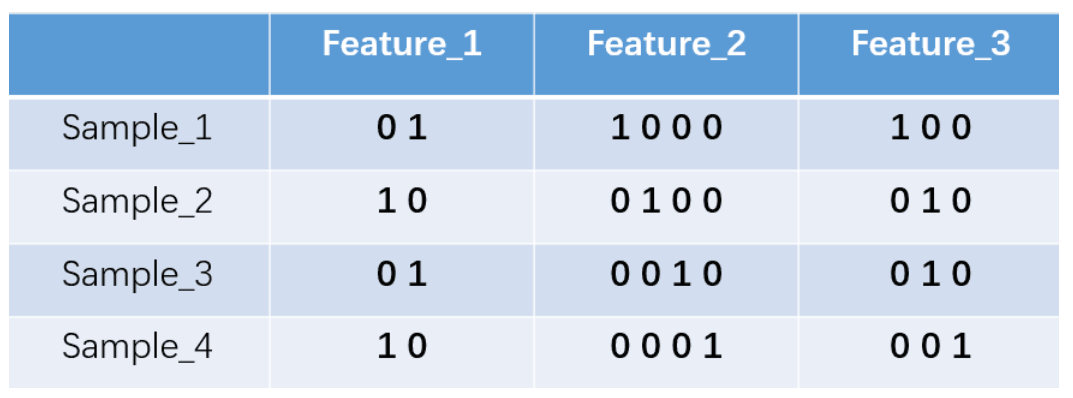如果将世界所有城市名称作为语料库的话，那这个向量会过于稀疏，并且会造成维度灾难。

Dristributed representation可以解决One hot representation的问题，它的思路是通过训练，将每个词都映射到一个较短的词向量上来。所有的这些词向量就构成了向量空间，进而可以用普通的统计学的方法来研究词与词之间的关系。这个较短的词向量维度是多大呢？这个一般需要我们在训练时自己来指定。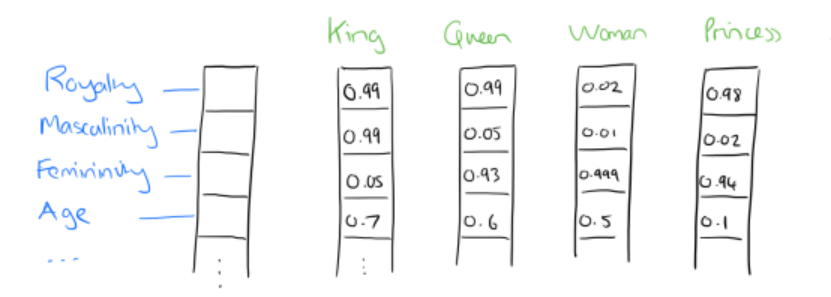（1）这个映射是单设（不懂的概念自行搜索）；
（2）映射之后的向量不会丢失之前的那种向量所含的信息。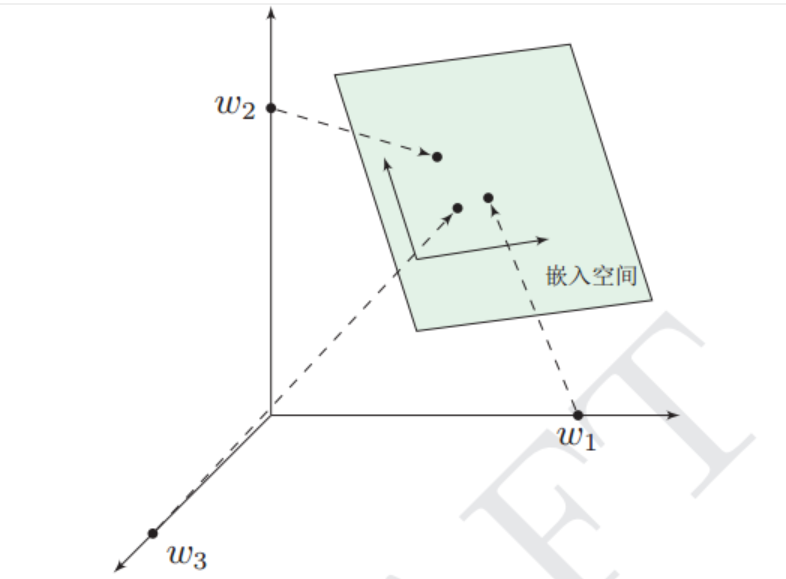经过我们一系列的降维神操作，有了用Dristributed representation表示的较短的词向量，我们就可以较容易的分析词之间的关系了，比如我们将词的维度降维到2维，有一个有趣的研究表明，用下图的词向量表示我们的词时，我们可以发现：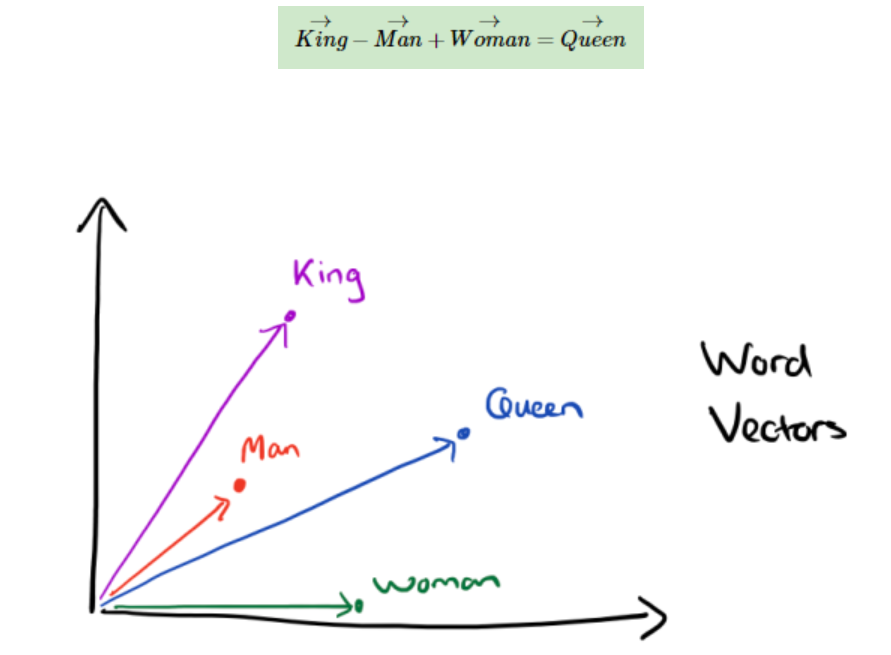哈哈哈

举个栗子：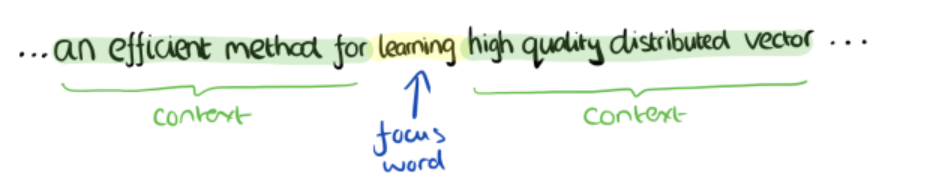# 2.word2vec

word2vec模型其实就是简单化的神经网络。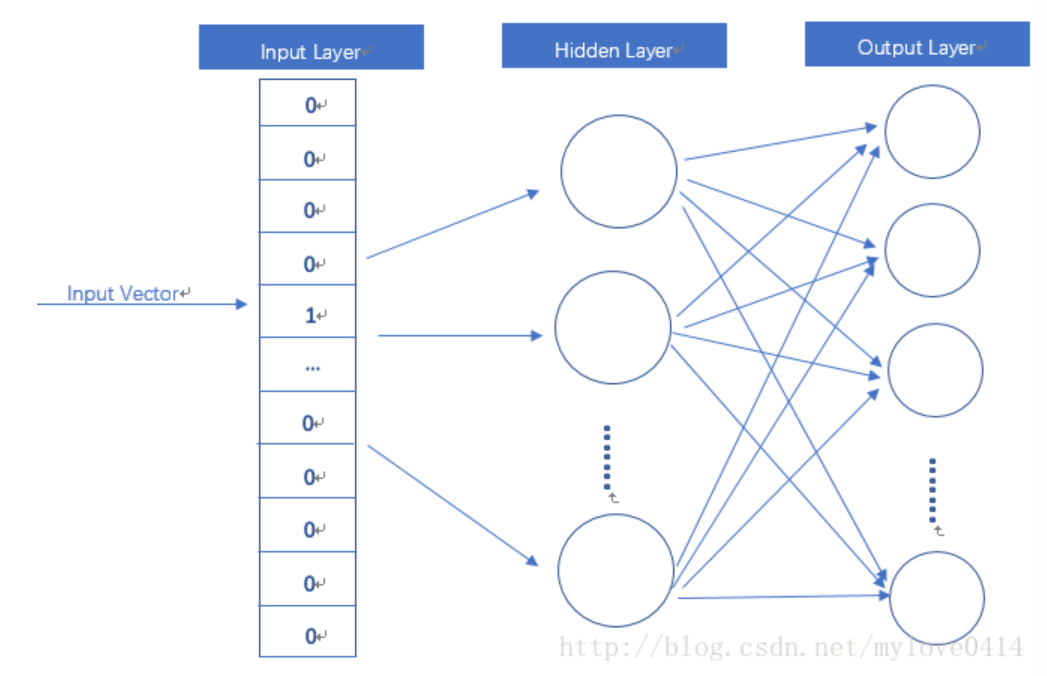输入是One-Hot Vector，Hidden Layer没有激活函数，也就是线性的单元。Output Layer维度跟Input Layer的维度一样，用的是Softmax回归。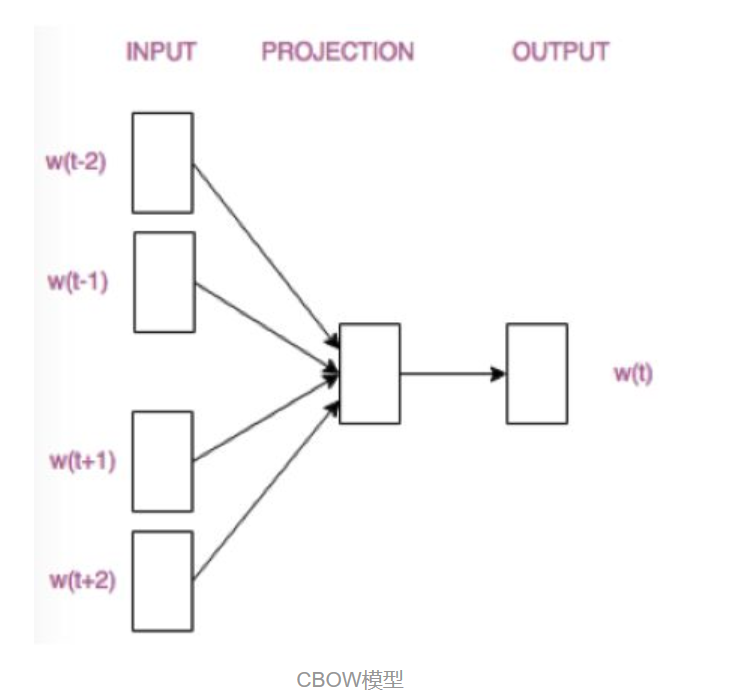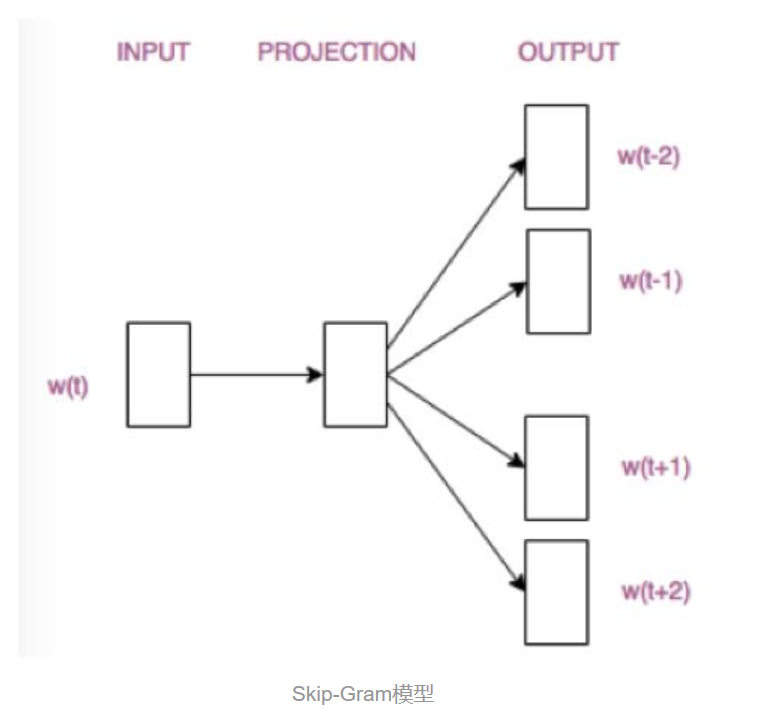## 2.1 CBOW(Continuous Bag-of-Words)

CBOW的训练模型如图所示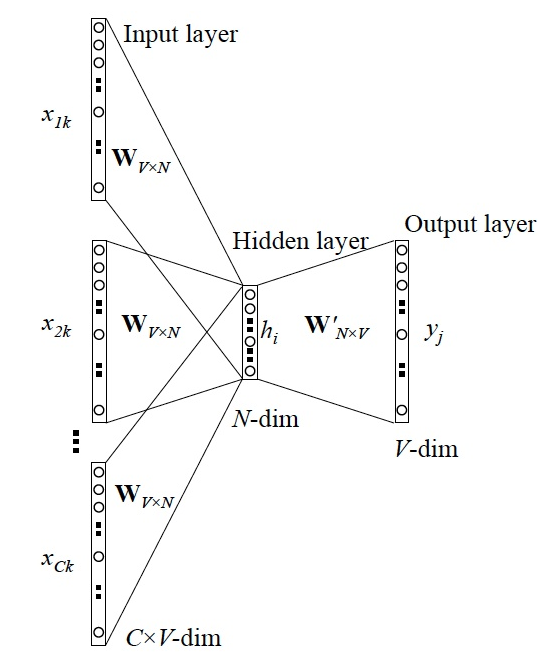1 输入层：上下文单词的onehot. {假设单词向量空间dim为V，上下文单词个数为C}
2 所有onehot分别乘以共享的输入权重矩阵W. {VN矩阵，N为自己设定的数，初始化权重矩阵W}
3 所得的向量 {因为是onehot所以为向量} 相加求平均作为隐层向量, size为1
N.
4 乘以输出权重矩阵W' {NV}
5 得到向量 {1
V} 激活函数处理得到V-dim概率分布 {PS: 因为是onehot嘛，其中的每一维斗代表着一个单词}
6 概率最大的index所指示的单词为预测出的中间词（target word）与true label的onehot做比较，误差越小越好（根据误差更新权重矩阵）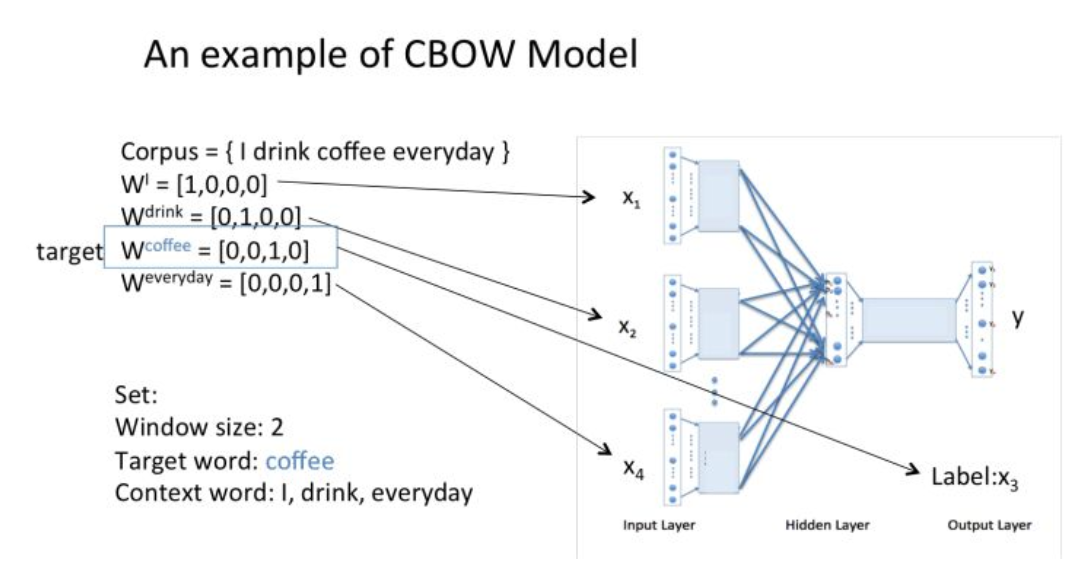窗口大小是2，表示选取coffe前面两个单词和后面两个单词，作为input词。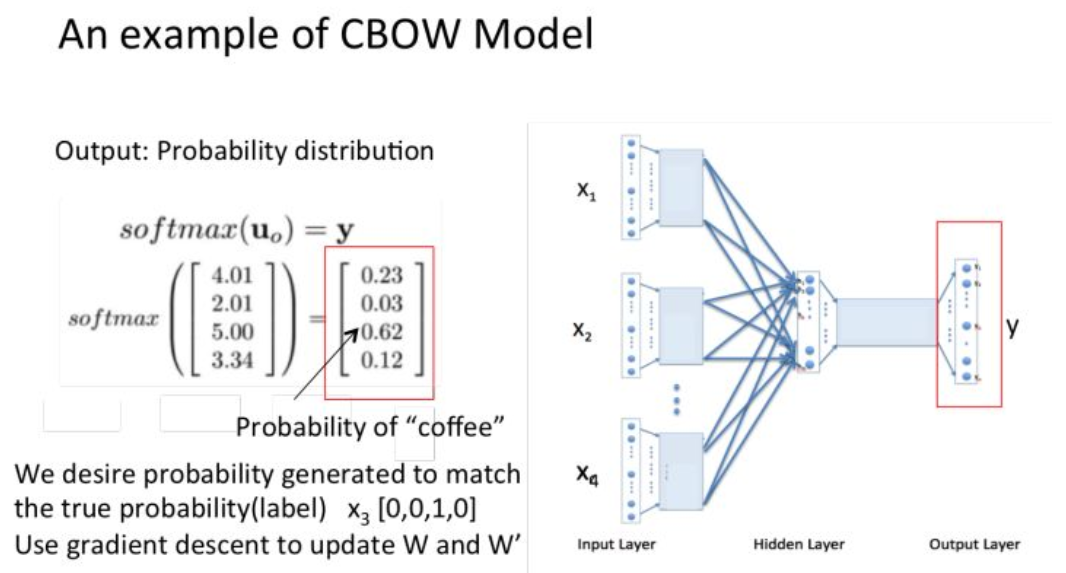假设我们此时得到的概率分布已经达到了设定的迭代次数，那么现在我们训练出来的look up table应该为矩阵W。即，任何一个单词的one-hot表示乘以这个矩阵都将得到自己的word embedding。

## 2.2 Skip-Gram

``````import tensorflow as tf
import matplotlib.pyplot as plt
import numpy as np

tf.reset_default_graph()

# 3 Words Sentence
sentences = [ "i like dog", "i like cat", "i like animal",
"dog cat animal", "apple cat dog like", "dog fish milk like",
"dog cat eyes like", "i like apple", "apple i hate",
"apple i movie book music like", "cat dog hate", "cat dog like"]

word_sequence = " ".join(sentences).split()
word_list = " ".join(sentences).split()
word_list = list(set(word_list))
word_dict = {w: i for i, w in enumerate(word_list)}

# Word2Vec Parameter
batch_size = 20
embedding_size = 2 # To show 2 dim embedding graph
voc_size = len(word_list)

def random_batch(data, size):
random_inputs = []
random_labels = []
random_index = np.random.choice(range(len(data)), size, replace=False)

for i in random_index:
random_inputs.append(np.eye(voc_size)[data[i]])  # target
random_labels.append(np.eye(voc_size)[data[i]])  # context word

return random_inputs, random_labels

# Make skip gram of one size window
skip_grams = []
for i in range(1, len(word_sequence) - 1):
target = word_dict[word_sequence[i]]
context = [word_dict[word_sequence[i - 1]], word_dict[word_sequence[i + 1]]]

for w in context:
skip_grams.append([target, w])

# Model
inputs = tf.placeholder(tf.float32, shape=[None, voc_size])
labels = tf.placeholder(tf.float32, shape=[None, voc_size])

# W and WT is not Traspose relationship
W = tf.Variable(tf.random_uniform([voc_size, embedding_size], -1.0, 1.0))
WT = tf.Variable(tf.random_uniform([embedding_size, voc_size], -1.0, 1.0))

hidden_layer = tf.matmul(inputs, W) # [batch_size, embedding_size]
output_layer = tf.matmul(hidden_layer, WT) # [batch_size, voc_size]

cost = tf.reduce_mean(tf.nn.softmax_cross_entropy_with_logits_v2(logits=output_layer, labels=labels))
optimizer = tf.train.AdamOptimizer(0.001).minimize(cost)

with tf.Session() as sess:
init = tf.global_variables_initializer()
sess.run(init)

for epoch in range(5000):
batch_inputs, batch_labels = random_batch(skip_grams, batch_size)
_, loss = sess.run([optimizer, cost], feed_dict={inputs: batch_inputs, labels: batch_labels})

if (epoch + 1)%1000 == 0:
print('Epoch:', '%04d' % (epoch + 1), 'cost =', '{:.6f}'.format(loss))

trained_embeddings = W.eval()

for i, label in enumerate(word_list):
x, y = trained_embeddings[i]
plt.scatter(x, y)
plt.annotate(label, xy=(x, y), xytext=(5, 2), textcoords='offset points', ha='right', va='bottom')
plt.show()``````

暂无相关的数据...